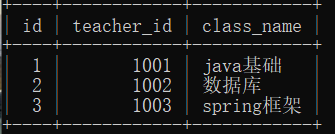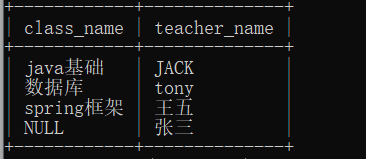WHCSRL 技术网

# Mysql知识点整合

1.库的操作

2.表的操作

3.基础函数

4.聚合函数

5.条件查询

6.关联查询

7.事务

8.索引

9.视图

10.总结

## 1.1建库（建demo）

``create database demo default character set utf-8;``

## 1.2删库（删demo）

``````drop database demo;
``````

## 1.3查库

``show databases;``

# 2.表的操作

## 2.1创建表

create table 表明 （字段名  字段类型 字段约束）；

``create table user (id int primary key auto_increment,name varchar(20),age int,sex varchar(10));``

## 2.2修改表

``alter table user add column tel int(30);``

## 2.5向表中插入数据

insert into 表名  values(值1，值2，值3，....值n)；

``insert into user values (null,'小明',18,'男',187633);``

``insert into user values (null,'小红',18,'女',187632);``

2.因为tel字段的约束设为了30，可能不足，可自行调节

## 2.6查询记录

select  要查询的字段  from  表名   条件;

``select * from user;``

``select * from user where sex='男';``

``select name from user where sex='男';``

## 2.7修改记录

update 表名 set 字段  条件；

``update user set name='张三' where id=1;``

## 2.8删除记录

delete from 表名 条件；

``delete from user where id=1;``

# 3.基础函数

``create table class (id int primary key auto_increment,teacher_id int,class_name varchar(50));``

``create table teacher (id int primary key auto_increment,teacher_id int,teacher_name varchar(20)dept_no int);``

``insert into class values(null,1001,'java基础');insert into class values(null,1002,'数据库');insert into class values(null,1003,'spring框架');````insert into teacher values(null,1001,'JACK',1);insert into teacher values(null,1002,'tony',1);insert into teacher values(null,1003,'王五',1);insert into teacher values(null,null,'张三',2);``

## 3.1 lower（数据转小写）

``select lower(teacher_name)from teacher where teacher_name='JACK';``

## 3.2 upper(数据转大写)

``select upper(teacher_name) from teacher where teacher_name='tony';``

## 3.3 length(查询数据的长度)

``select length(teacher_name) from teacher where id=3;``

## 3.4 substr

``select substr(class_name,1,3) from class where id=1;``

## 3.5 contat（拼接数据）

``select concat(class_name,'大全') from class where id=1;``

## 3.6 replace（替换）

``select replace(teacher_name,'J','c') from teacher where id=1;``

## 3.7 ifnull(判断是否为null ，若为null 则用***替换)

``select ifnull(teacher_id,1004) from teacher where id=4;``

## 3.9 时间

select now() -- 年与日 时分秒

select curdate() --年与日

select curtime() --时分秒

### year & month & day

–hour()时 minute()分 second()秒

select now(),hour(now()),minute(now()),second(now()) from emp ;

–year()年 month()月 day()日

select now(),year(now()),month(now()),day(now()) from emp ;

# 4.聚合函数

## 4.1 count（数量）

``select count(*) from class;  //方法一：底层优化过select count(1) from class;  //方法二：与方法一效果一样select count(id) from class; //方法三：慢，只能统计非null的``

## 4.2max（最大值）、min（最小值）

``select max(teacher_id) max,min(teacher_id) min from class;``

## 4.3 sum(总和)、avg（平均数）

``select sum(teacher_id) sum ,avg(teacher_id) avg from teacher;``

# 5.条件查询

## 5.1 distinct去重

``select distinct dept_no from teacher;``

## 5.2 where(条件)

``select teacher_name from teacher where id=1;``

## 5.3 like（模糊查询）

``select * from teacher where teacher_name like 'J%%'; --以J开头的 select * from teacher where teacher_name like '%%y'; --以y结束的 select * from teacher where teacher_name like '%%A%%'; --中间包含A的 select * from teacher where teacher_name like 'o__'; --o后面有两个字符的 _代表一个字符位置``

## 5.4 null

``select * from teacher where teacher_id is null; --过滤字段值为空的 select * from teacher where teacher_id is not null; --过滤字段值不为空的``

## 5.5 between and

``select * from teacher where teacher_id <1002 and teacher_id>1000; select * from teacher where teacher_id<=1002 and teacher_id>=1000; select * from teacher where teacher_id between 1000 and 1002;``

## 5.6 limit （分页）

``select * from teacher limit 2 ;--列出前两条 select * from teacher limit 1,2; --从第二条开始,展示2条记录 select * from teacher limit 0,3; --从第一条开始,展示3条记录--前三条``

## 5.7 order by（排序）

``SELECT * FROM teacher order by teacher_id ;#默认升序 SELECT * FROM teacher order by teacher_id desc; #降序``

## 5.8 group by（分组）

``select max(teacher_id) max,dept_no from teachergroup by dept_no;``

## 5.9 having（条件）

having与where类似，但是group by只能跟having连用，且having用于聚合函数；

``select max(teacher_id) max ,dept_nofrom teacher group by dept_nohaving max>1000;``

# 6.关联查询(多表联查)

## 6.1笛卡尔积

``select * from teacher,class;``

## 6.2 join

### 内连接  join（inner join）

``select c.class_name,t.teacher_name from class c join teacher ton c.teacher_id=t.teacher_id;``

### 左连接 left join

``select c.class_name,t.teacher_name from class c left join teacher ton c.teacher_id=t.teacher_id;``

### 右连接 right join

``select c.class_name,t.teacher_name from class c right join teacher ton c.teacher_id=t.teacher_id;``总结：

1.使用内连接，不会打印null值，而使用外连接会填充null值。

2.使用内连接，是取两张表的交集，同理三表联查的话就是三张表的交集；

## 6.3 子查询

``select class_name from classwhere teacher_id in (select teacher_id from teacherwhere teacher_name='王五');``

(1) FROM [left_table] 选择表

(2) ON <join_condition> 链接条件

(3) <join_type> JOIN <right_table> 链接

(4) WHERE <where_condition> 条件过滤

(5) GROUP BY <group_by_list> 分组

(6) AGG_FUNC(column or expression),... 聚合

(7) HAVING <having_condition> 分组过滤

(8) SELECT (9) DISTINCT column,... 选择字段、去重

(9) ORDER BY <order_by_list> 排序

(10) LIMIT count OFFSET count; 分页

# 8.索引

## 8.1分类

• 单值索引：一个索引只包括一个列，一个表可以有多个列
• 唯一索引：索引列的值必须唯一，但允许有空值；主键会自动创建唯一索引
• 复合索引：一个索引同时包括多列

## 8.2 创建索引

### 查看索引

``show index from class;``

### 创建普通索引

create index 索引名字 on 表名(字段名);

``create index cla_index on class(teacher_id);``

### 创建唯一索引

CREATE UNIQUE INDEX 索引名 ON 表名(字段名);

``create unique index class_index on class(teacher_id);``

### 创建复合索引

CREATE INDEX 索引名 ON 表名 (字段1, 字段2)

``create index tea_index on teacher(teacher_id,teacher_name);``

### 删除索引

``alter table teacher drop index tea_index;``

### 索引的扫描类型

ALL 全表扫描，没有优化，最慢的方式
index 索引全扫描，其次慢的方式
range 索引范围扫描，常用语<，<=，>=，between等操作
ref 使用非唯一索引扫描或唯一索引前缀扫描，返回单条记录，常出现在关联查询中
eq_ref 类似ref，区别在于使用的是唯一索引，使用主键的关联查询
const/system 单条记录，系统会把匹配行中的其他列作为常数处理，如主键或唯一索引查询，system是const的特殊情况
null MySQL不访问任何表或索引，直接返回结果

### 索引优缺点

1.索引是数据库优化
2.表的主键会默认自动创建索引
3.每个字段都可以被索引
4.大量降低数据库的IO磁盘读写成本，极大提高了检索速度
5.索引事先对数据进行了排序，大大提高了查询效率

1.索引本身也是一张表，该表保存了主键与索引字段，并指向实体表的记录，所以索引列也要占用空间
2.索引表中的内容，在业务表中都有，数据是重复的，空间是“浪费的”
3.虽然索引大大提高了查询的速度，但对数据的增、删、改的操作需要更新索引表信息，如果数据量非常巨大，更新效率就很慢,因为更新表时,MySQL不仅要保存数据,也要保存一下索引文件
4.随着业务的不断变化，之前建立的索引可能不能满足查询需求，需要消耗我们的时间去更新索引

## 9.视图

可视化的表，视图当做是一个特殊的表，是指，把sql执行的结果，直接缓存到了视图中。

## 创建、使用视图

create view 视图名 as SQL语句;

select * from 视图名;

`` //创建 视图create view viewdemo asselect *from class ; //使用 视图sele* from viewdemo;``

#视图：就是一个特殊的表，缓存上次的查询结果

#好处是提高了SQL的复用率，坏处是占内存无法被优化